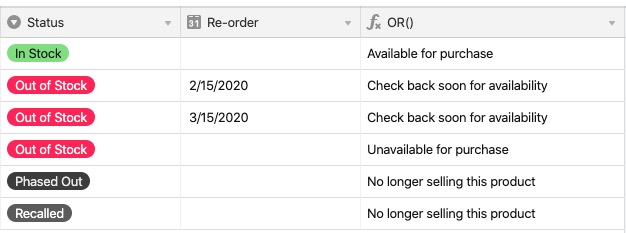• English
• Account
• API
• Integrations
• Enterprise
• Resources
Evaluate arguments using AND() and OR()
• 03 Mar 2023
• Dark
Light
• PDF

# Evaluate arguments using AND() and OR()

• Dark
Light
• PDF

Article Summary

In this article, we'll primarily be working with two very similar formulas:
`AND()` and `OR()`. This article is part of a guided course which you can view here.

FunctionBehaviorUse caseStructure
`AND()`
Returns true if all the arguments are true, returns false otherwise.
Using `AND()`, you can list out any number of criteria to evaluate. If they are all true, the function will return a 1 (true), and if not all are true, it will return a 0 (false).
`AND(logical 1, logical 2, logical 3, etc...)`
`OR()`
Returns true if any one of the arguments is true, returns false otherwise.
Using `OR()`, you can list out any number of criteria to evaluate. If any of the values are true, the function will return a 1 (true), and if not all are true, it will return a 0 (false).
`OR(logical 1, logical 2, logical 3, etc...)`

## Working with `AND()`

`AND ()`can be used on it's own as a standalone function, and it can also be used in conjunction with conditional statements.

As an example of using the function on it's own, let's write a formula to check if the following fields have a value in them:

•  Status
•  Project Owner
•  Due Date

Regardless of what function you're using, you can reference a field name to see if it has anything entered in it. For example, to check if a {Status} field has a value, you can just write: IF({Status}....

``````AND(
{Status},
{Project Owner},
{Due Date}
)``````

If all three fields have any value in them, the formula will return a 1, otherwise a 0.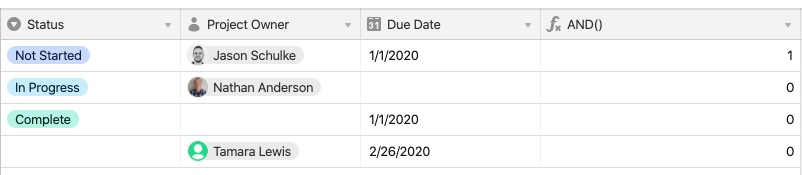Notice that only the first record has a value of `1` because all three fields referenced in the formula have something entered in the field.

## Using `AND () `with conditional statements

Taking the function a step further, let's see what it looks like to nest it within another statement. Using the same table structure, you have the following criteria:

• Projects that are assigned to Nathan
•  And projects that are in progress
•  Should show the message, "Nathan - Active"

Using `AND()`, here is the formula you would write:

``````IF(
AND(
{Project Owner} =  "Nathan Anderson",
Status = "In Progress"
),
"Nathan - Active"
)``````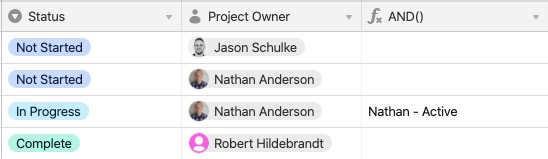Let's change the criteria to look for the following:

• For projects that are not started
• And projects without a project owner
• Show a message, "Needs Owner"
• Otherwise, show "Next in Queue"

Here’s the formula:

``````
IF (
AND (
{Status} = "Not Started" ,
{Project Owner}
),
"Next in Queue" ,
"Needs Owner"
)

``````

And here’s the result: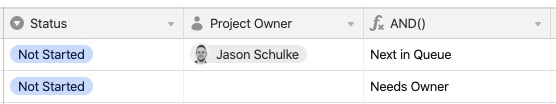Let's build in some more complex conditions using `AND()`

• For projects that are not started
• And projects with a project owner
• Show a message, "Next in Queue"
• Otherwise, show "Needs Owner"
• And for projects in progress, show "Due Soon"
• Otherwise, for complete projects show "Complete"

Here’s the formula:

``````IF(
AND(
{Status} = "Not Started",
{Project Owner}
),
"Next in Queue",

IF(
AND(
{Status} = "Not Started",
{Project Owner}=BLANK()
),
"Needs Owner",

IF(
AND(
{Status} = "In Progress",
{Due Date}
),
"Due soon",
"Complete"
)
)
)``````

And the result: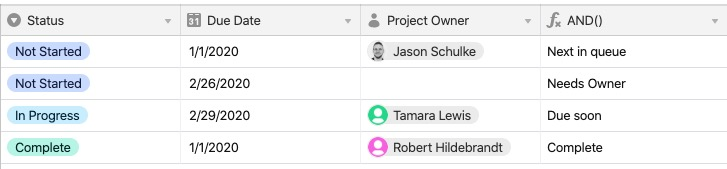The formula above is not complete, as it doesn't account for all potential scenarios when some of the field values are empty. It's only meant to show a portion of the various
`AND() `functions and their usage.

## Working with `OR()`

The` OR() `function can also be used alone, or in combination with other functions.

Remember that when used by itself, `OR()`produces either a `1`or `a `depending if *any* of the criteria listed in the statement are true. For example, `OR (5+5=10, 5+5=12) `will evaluate as `1` because one of the statements is true.

As an example, you want to display a message depending on the quantity of available items you have in stock. Let's start with the function by itself:

``````OR(
{Status} = "Out of Stock",
{Status} = "Delayed Backorder"
)``````

Here’s the result: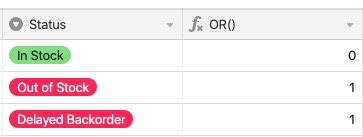Using `OR()` with conditional statements

Building on the previous section, you may now want to display a message depending on if the result is a 1 or 0. You want to show "Available for purchase" if the item is in stock, or "Currently unavailable" if it is out of stock or on delayed backorder.

``````IF(
OR(
{Status} = "Out of Stock",
{Status} = "Phased Out"
),
"Currently unavailable",
"Available for purchase"
)``````

Here is the example result for that formula: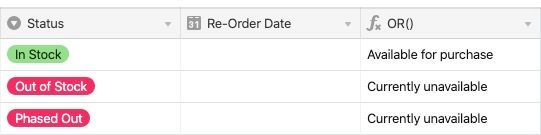## Combining `AND()``+ OR()`

Here’s an example of combining `AND() `and `OR() `together.

``````IF(
Status = "In Stock",
"Available for purchase",

IF(
AND(
Status = "Out of Stock",
{Re-order}
),
"Check back soon for availability",

IF(
OR(
Status = "Phased Out",
Status = "Recalled"
),
"No longer selling this product",
"Unavailable for purchase"
)
)
)``````

And here’s the result you can expect: